# 一篇文章教会你使用Python下载抖音无水印视频

sublime text 3、360浏览器、Python 3.6运行环境

``多少英雄豪杰被黑，只为神化一个痴呆患者李元霸 https://v.douyin.com/wCgKrh/ 复制此链接，打开【抖音短视频】，直接观看视频！``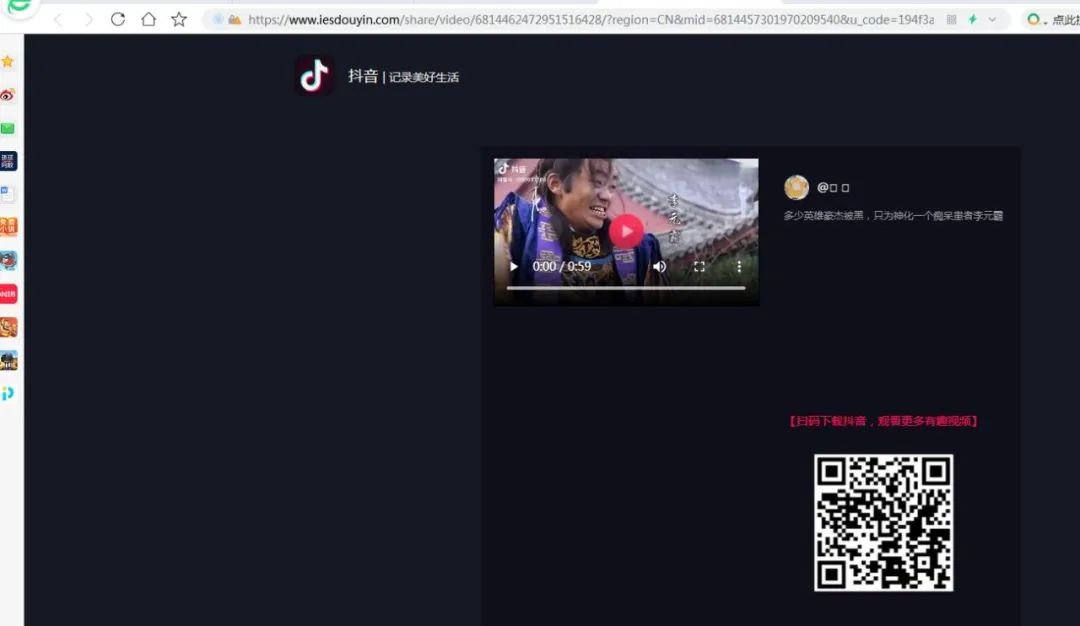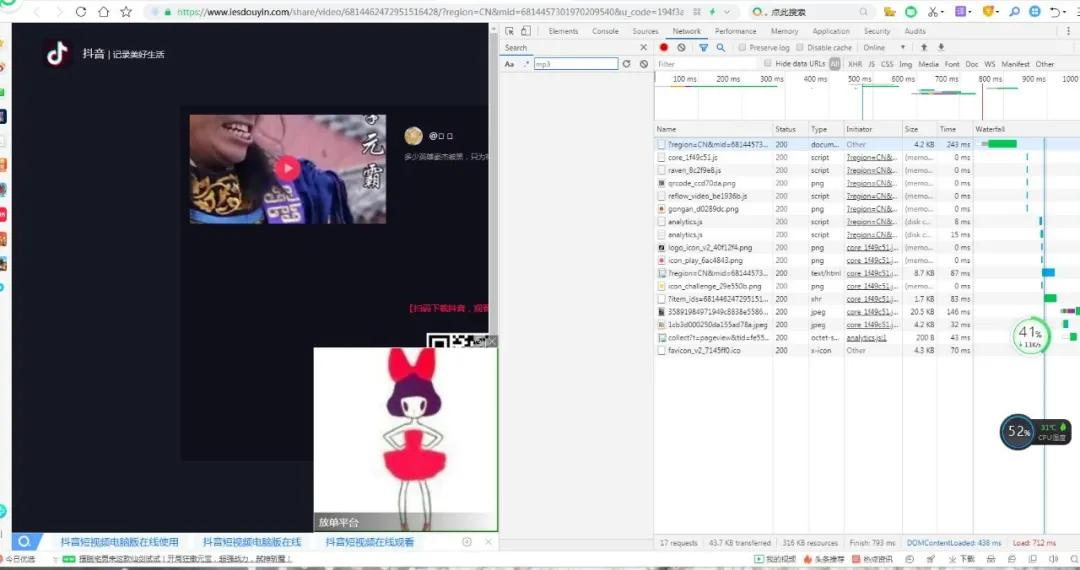``````class page:
def __init__(self):
self.root= tk.Tk()   #初始化窗口
self.root.title('抖音无水印视频下载v1.0')  #窗口名称
self.root.geometry("700x700")  #设置窗口大小
#设置窗口是否可变，宽不可变，高可变，默认为True
self.root.resizable(width=True,height=True)
#创建标签,文字，背景颜色，字体（颜色，大小），标签的高和宽
self.label1 =tk.Label(self.root,text='抖音地址：',font=('宋体',10),width=12,height=2)
#创建输入框，标签高度，字体大小颜色，内容显示方式
self.e1 = tk.Entry(self.root,width=30,show=None, font=('Arial', 12))
self.label2 =tk.Label(self.root,text='文件名：',font=('宋体',10),width=12,height=2)
#创建输入框，标签高度，字体大小颜色，内容显示方式
self.e2 = tk.Entry(self.root,width=30,show=None, font=('Arial', 12))
#创建按钮 内容  宽高  按钮绑定事件
self.b2 = tk.Button(self.root, text='清空内容', width=8,height=1,command=self.close)
self.photo=tk.PhotoImage(file = '111.gif')
self.im=tk.Label(self.root, image = self.photo)
self.photo1=tk.PhotoImage(file = '222.gif')
self.im1=tk.Label(self.root, image = self.photo1)
self.dashang=tk.Label(self.root,bg='gray',fg='blue',font=('宋体',12),text='感谢各位的支持，觉得小弟写的不错欢迎打赏，以便于我日后分享更多的精彩作品')
#将所有部件添加到界面中
self.label1.place(x=140,y=30,anchor='nw')
self.e1.place(x=210,y=32,anchor='nw')
self.b2.place(x=500,y=40,anchor='nw')
self.label2.place(x=144,y=60,anchor='nw')
self.e2.place(x=210,y=62,anchor='nw')
self.b1.place(x=230,y=110,anchor='nw')
self.dashang.place(x=60,y=160)
self.im.place(x=10,y=200,width=202,height=313,anchor='nw')
self.im1.place(x=300,y=200,width=202,height=313,anchor='nw')
self.b3=tk.Button(self.root,text='技术博客，点我直达',command=self.refer)
self.b3.place(x=200,y=530,width=140,height=40,anchor='nw')
self.root.mainloop()``````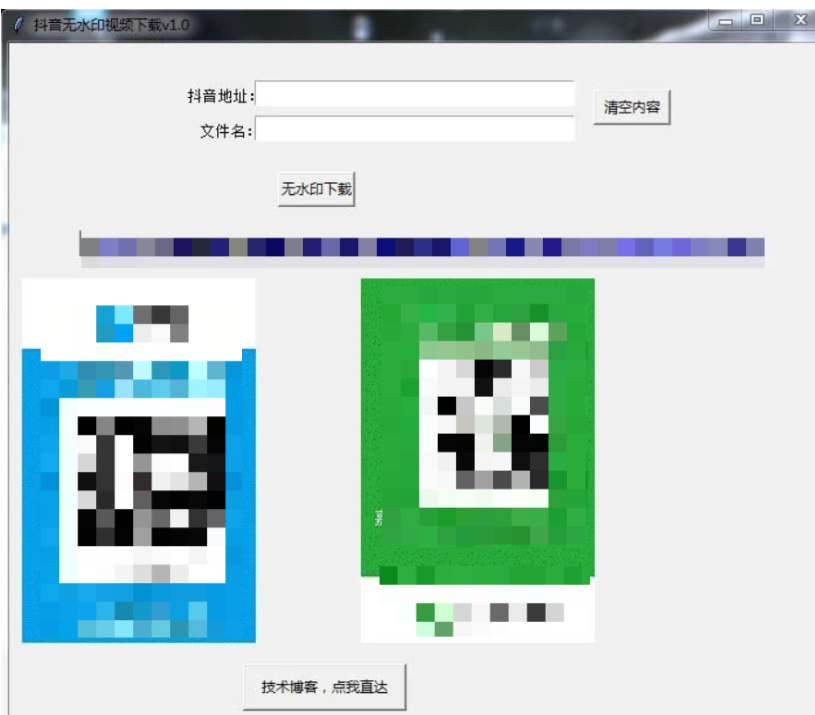``````def download(self):
url='http://www.zimo.wiki:8080/douyin-video-crawler/api/analysis?url='+self.e1.get()
try:
rep=requests.get(url,timeout=5)
result=rep.text
res=eval(result)
if res.get('msg')=='analysis success':
down_url=res.get('url')
data=requests.get(down_url,stream=True,timeout=4)
with open('{}.mp4'.format(self.e2.get()),'wb') as f:
f.write(data.content)
else:
msg.showwarning('notice','URL Format Error!!!')
except:
return``````

``http://www.zimo.wiki:8080/douyin-video-crawler/api/analysis?url=``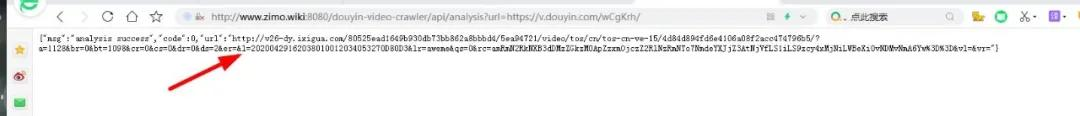``````def close(self):  #关闭
self.e1.delete(0,'end')
self.e2.delete(0,'end')

def refer(self): #跳转
wb.open('https://url.ms/lypks')``````

``page()``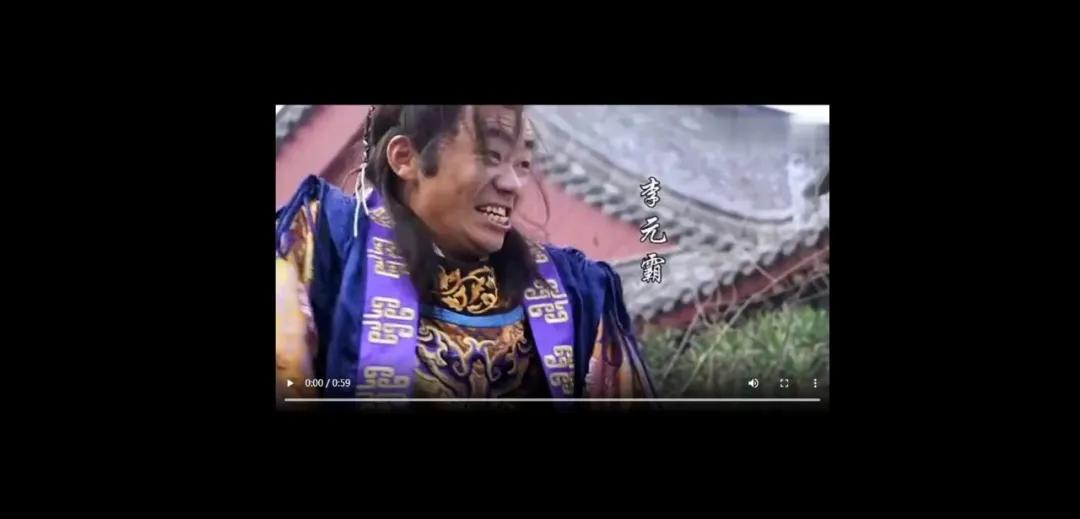IT共享之家——————- End ——————-